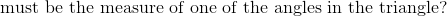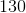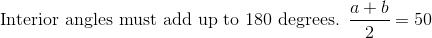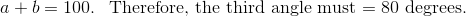# PSAT Math : How to find an angle in an acute / obtuse triangle

## Example Questions

### Example Question #11 : How To Find An Angle In An Acute / Obtuse Triangle

If the average of the measures of two angles in a triangle is 75o, what is the measure of the third angle in this triangle?

65°

75°

50°

30°

40°

30°

Explanation:

The sum of the angles in a triangle is 180o:  a + b + c = 180

In this case, the average of a and b is 75:

(a + b)/2 = 75, then multiply both sides by 2

(a + b) = 150, then substitute into first equation

150 + c = 180

c = 30

### Example Question #12 : How To Find An Angle In An Acute / Obtuse Triangle

Which of the following can NOT be the angles of a triangle?

45, 90, 100

30.5, 40.1, 109.4

1, 2, 177

45, 45, 90

30, 60, 90

45, 90, 100

Explanation:

In a triangle, there can only be one obtuse angle. Additionally, all the angle measures must add up to 180.

### Example Question #13 : How To Find An Angle In An Acute / Obtuse Triangle

Let the measures, in degrees, of the three angles of a triangle be x, y, and z. If y = 2z, and z = 0.5x - 30, then what is the measure, in degrees, of the largest angle in the triangle?

96
30
48
60
108
Explanation:

The measures of the three angles are x, y, and z. Because the sum of the measures of the angles in any triangle must be 180 degrees, we know that x + y + z = 180. We can use this equation, along with the other two equations given, to form this system of equations:

x + y + z = 180

y = 2z

z = 0.5x - 30

If we can solve for both y and x in terms of z, then we can substitute these values into the first equation and create an equation with only one variable.

Because we are told already that y = 2z, we alreay have the value of y in terms of z.

We must solve the equation z = 0.5x - 30 for x in terms of z.

z + 30 = 0.5x

Mutliply both sides by 2

2(z + 30) = 2z + 60 = x

x = 2z + 60

Now we have the values of x and y in terms of z. Let's substitute these values for x and y into the equation x + y + z = 180.

(2z + 60) + 2z + z = 180

5z + 60 = 180

5z = 120

z = 24

Because y = 2z, we know that y = 2(24) = 48. We also determined earlier that x = 2z + 60, so x = 2(24) + 60 = 108.

Thus, the measures of the three angles of the triangle are 24, 48, and 108. The question asks for the largest of these measures, which is 108.

### Example Question #14 : How To Find An Angle In An Acute / Obtuse Triangle

Angles x, y, and z make up the interior angles of a scalene triangle. Angle x is three times the size of y and 1/2 the size of z. How big is angle y.

36

108

54

42

18

18

Explanation:

We know that the sum of all the angles is 180. Using the rest of the information given we can write the other two equations:

x + y + z = 180

x = 3y

2x = z

We can solve for y and z in the second and third equations and then plug into the first to solve.

x + (1/3)x + 2x = 180

3[x + (1/3)x + 2x = 180]

3x + x + 6x = 540

10x = 540

x = 54

y = 18

z = 108

### Example Question #1 : How To Find An Angle In An Acute / Obtuse Triangle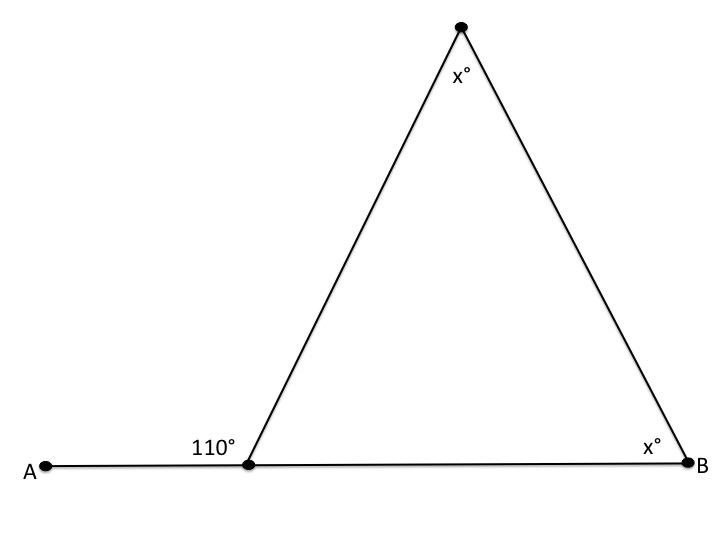In the picture above,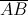is a straight line segment. Find the value of.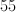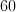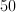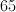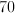Explanation:

A straight line segment has 180 degrees. Therefore, the angle that is not labelled must have: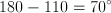We know that the sum of the angles in a triangle is 180 degrees. As a result, we can set up the following algebraic equation: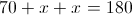Subtract 70 from both sides: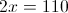Divide by 2: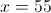### Example Question #1 : How To Find An Angle In An Acute / Obtuse Triangle

An exterior angle of an isosceles triangle measures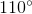. What is the least measure of any of the three interior angles of the triangle?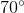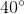Insufficient information is given to answer this question.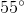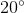Insufficient information is given to answer this question.

Explanation:

The triangle has an exterior angle of, so it has an interior angle of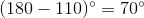. By the Isosceles Triangle Theorem, an isosceles triangle must have two congruent angles; there are two possible scenarios that fit this criterion:

I: One of the other angles also has measure.

In this case, since the angles' measures must totalthe third angle has measure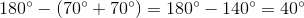In this case, the least measure is.

II: The other two angles are the congruent angles.

In this case, the other two angles have measures totaling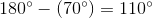.

They have the same measure, so each has measure half this, or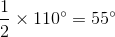.

In this case, the least measure isTherefore, insufficient information is given to answer this question.

### Example Question #2 : How To Find An Angle In An Acute / Obtuse Triangle

An exterior angle of an isosceles triangle measures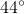. What is the greatest measure of any of the three angles of the triangle?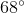Insufficient information is given to answer this question.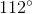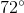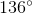Explanation:

The triangle has an exterior angle of, so it has an interior angle of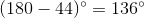. This is an obtuse angle; the other two angles must be acute, and therefore, they will have measure less than- and, subsequently, theangle will be the one of greatest measure.

### Example Question #3 : How To Find An Angle In An Acute / Obtuse Triangle